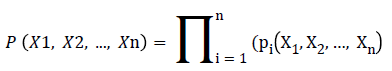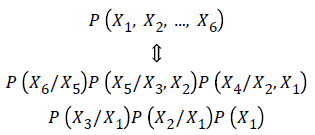## Biomedical Research

All submissions of the EM system will be redirected to Online Manuscript Submission System. Authors are requested to submit articles directly to Online Manuscript Submission System of respective journal.

Research Article - Biomedical Research (2018) Artificial Intelligent Techniques for Bio Medical Signal Processing: Edition-II

## A novel segmentation method of medical CBCT image in liver organ based on Bayesian network

Huiling Guo1, Haizhi Hu1 and Guobing Fan2*

1College of Computer Science and Technology, Zhoukou Normal University, Henan, Zhoukou, PR China

2Department of Basic Subjects, Hunan University of Finance and Economics, Hunan, Changsha, PR China

*Corresponding Author:
Guobing Fan
Department of Basic Subjects
Hunan University of Finance and Economics, PR China

Accepted date: June 24, 2017

Visit for more related articles at Biomedical Research

### Abstract

Introduction: This paper proposed a kind of segmentation method for medical CBCT image in liver organ based on Bayesian network.

Methods: Taking CBCT medical image of liver organ as a focus of medical image segmentation and to segment the area we are interested in with noise elimination and image enhancement. In order to show multiple image information including the liver organ CBCT image color, texture, shape and other features, image information can be extracted, and the extracted image features can be classified from the perspective of data mining by Bayesian network.

Results: The method ensures that the target points of the liver organ CBCT image are clearly visible, which is not only suitable for the normal image registration, but also for the disordered image registration. Bayesian network method has good organization ability to distinguish pixels with similar gray level belonging to different tissues in the image, and map them back to the image space, so that tissue extraction from liver organ CBCT images has a good effect.

Conclusion: The CBCT images are analysed based on medical image database to better achieve the required characteristics in data mining, extraction of non-rigid registration and image segmentation technology. And the result shows that this kind of method based on Bayesian network is a good theoretical method that can achieve expected outcome and the accuracy rate is good with high practical application.

## Keywords

Medical CBCT image, Liver organ, Segmentation method, Bayesian network, Probability

## Introduction

Medical image segmentation is to separate an image into multiple regions with similar characteristics, and propose new technology and processing based on the area that we are interested. This is an important method for researchers working in medical image processing and analyzing [1,2]. The focus of medical image segmentation is to segment the area we are interested in, such as the lesion area, tumor, etc., and make our segmentation result closing enough to anatomical structure, so as to help the clinicians analyzing patients’ condition, and making diagnosis and treatment correctly. Although there are many different segmentation methods, we have not yet developed an effective segmentation method which can satisfy the clinical demands [3,4]. Due to the difference of individual liver and structures, combined with the lesion area worse a lot more, the clinical algorithm demands higher requirements on medical image segmentation accuracy. On the other hand, the image noise and artifacts affect the image analyzing, making the existing image segmentation algorithms far away from the clinical demands [5,6].

## Methodology

A Bayesian network is a Directed Acyclic Graph  (DAG) that is composed by representative variable nodes and directed edges. The directed edge uses the parent nodes to point to the descendant nodes. In Bayesian network, there are some nodes called root nodes. They have the prior probability distribution which is associated with them and that can be directly provided. The non-root nodes are called child nodes. The source is known as parent nodes and each child node has a conditional probability distribution of the parent node under value state. Each node in Bayesian network has a Conditional Probability Table (CPT) [8,9].

If A represents the node variable and B1, B2, ..., Bn refers to the parent node of A. For node A, the CPT table refers to the value of P (A|B1, B2, ...., Bn). The correlation degree between nodes is characterized by the conditional probability value. The conditional probability table of each variable is calculated by the value of its parent node. For the variable without parent nodes, it can be obtained by the historical statistics as well as the knowledge and the experience of experts. A simple Bayesian network diagram is shown in Figure 1.

When the network diagram has the relationships and the conditional probability tables between nodes, the Bayesian network can express the joint probability of all nodes in network. Meanwhile, the network can calculate the probability information in any node according to the prior probability information and the values of some nodes. The joint probability P (X1, X2, ..., Xn) of Bayesian network is shown in followed formula.Through the above formula, the distribution function of joint probability of Bayesian network is shown in followed formula.For the construction of Bayesian network, there are five steps:

Step 1. Determining the nodes, namely determining the target nodes of research questions;

Step 2. Determining the relationships between nodes;

Step 3. Connecting with related nodes and constructing Bayesian network diagram;

Step 4. Assigning the nodes in constructed network diagram;

Step 5. Reckoning the Bayesian network according to the relationships between nodes and the mathematical nature of Bayesian network.

## Results

The range of the set probability is (0, 1) and the value of all pixels represents the probability belonging to the liver. As shown in the Figure 2.

Because the liver lesions have large difference in shape, we use a sparse linear combination optimization to solve the over segmentation or under segmentation will be combined with the sparse shape and probability map, through TV-L1 preprocessing, the segmentation result was obtained from liver is shown in Figure 3.

The results show that using SIFT to automatically match the feature points can effectively improve the segmentation results of sparse shape. It is proved that the segmentation of liver CT image can be improved by combining the probability map and the shape model.

## Conclusion

Medical images included abundant characteristic information have been divided into data’s characteristic mining and denoising processed data analysis by data mining and computer technology, and the characteristic extraction based on Bayesian network analysis is obtained, and image data will be further analysed by de-noising processed images. The segmentation method proposed in this paper can be applied to CBCT-based image-guided radiation system and PET. CT imaging system, can improve the accuracy of radiation therapy, and improve the efficiency of clinicians and oncologists.

## Acknowledgment

This work was supported by the Natural Science Foundation of Hunan Province, China (Grant No.2016JJ4012).